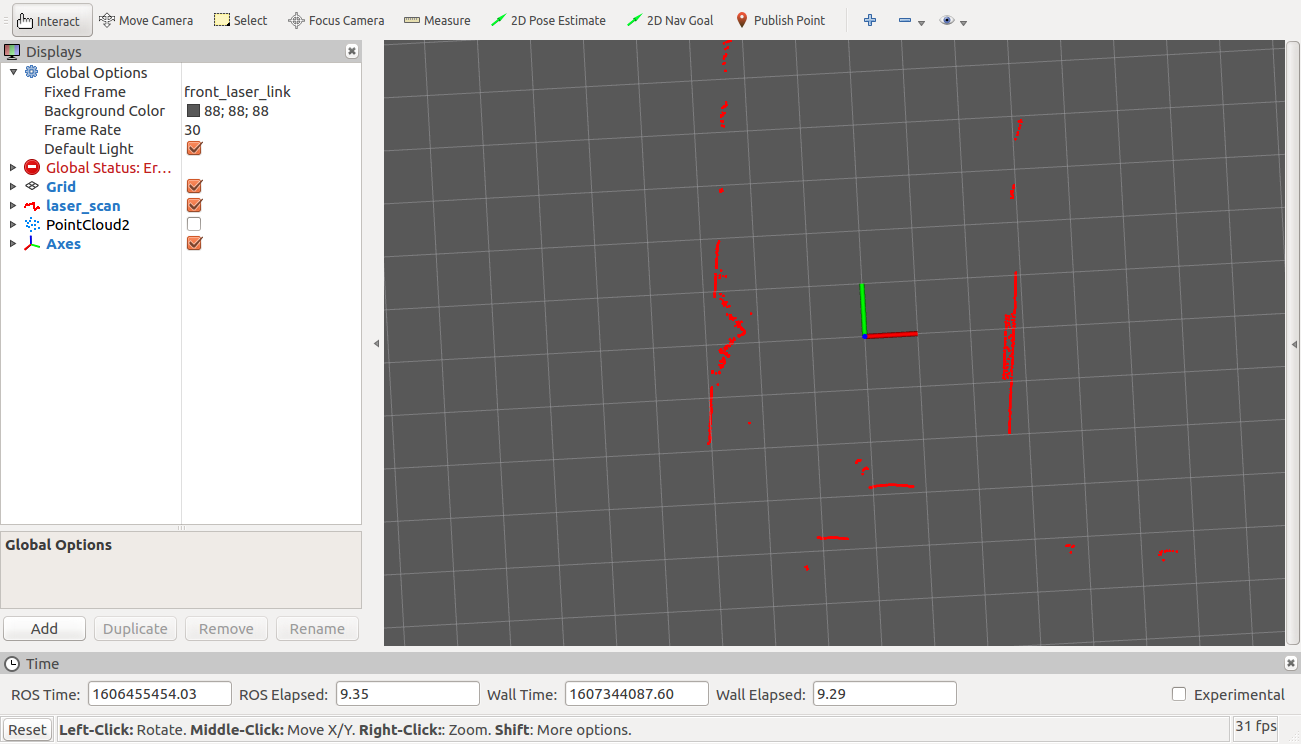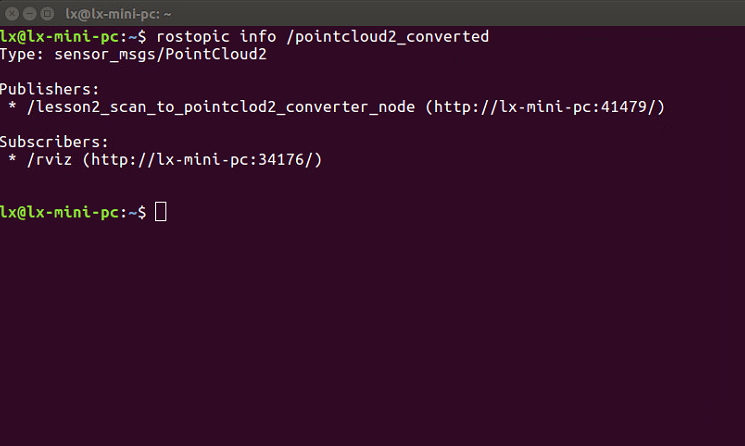# 1 PCL

PCL（Point Cloud Library）作为我们接触的第一个外部库，可见其重要性。

PCL里实现了大量的处理点云相关的功能，实现了大量点云相关的通用算法和高效数据结构，涉及到点云获取、滤波、分割、配准、检索、特征提取、识别、追踪、曲面重建、可视化等。

# 2 在ros中使用PCL

## 2.1 新生成包

cd ~/catkin_ws/src/Creating-2D-laser-slam-from-scratch
catkin_create_pkg lesson2 pcl_conversions pcl_ros roscpp sensor_msgs


roscpp以及sensor_msgs在之前的文章说过了，roscpp是c++的依赖，sensor_msgs是雷达数据的消息类型的依赖。

pcl_conversions 以及 pcl_ros 是ROS官方为了在ROS中方便的使用PCL而写的包。

pcl_conversions 包含了一些方法，实现了 ROS的消息类型 与 PCL的消息类型 的转换。

pcl_ros 定义了一些其他的功能，如在ROS中使用标准的Publisher直接发布PCL的数据格式、将PCL的点云根据tf进行坐标变换、实现了一些常用的功能接口，使得可以直接在ROS中调用PCL的函数，例如进行体素滤波等等。

https://blog.csdn.net/tiancailx/article/details/110816649

## 2.2 修改CMakeLists.txt

find_package(PCL REQUIRED QUIET)
include_directories(
include
${catkin_INCLUDE_DIRS}${PCL_INCLUDE_DIRS}
)


## 2.3 修改Package.xml

<build_depend>libpcl-all-dev</build_depend>
<exec_depend>libpcl-all</exec_depend>


# 3 工程代码

## 3.1 头文件

#ifndef LESSON2_SCAN_TO_POINTCLOUD2_CONVERTER_H
#define LESSON2_SCAN_TO_POINTCLOUD2_CONVERTER_H

// ros
#include <ros/ros.h>
#include <sensor_msgs/LaserScan.h>

// pcl_ros
#include <pcl_ros/point_cloud.h>

// pcl
#include <pcl/point_cloud.h>
#include <pcl/point_types.h>

class ScanToPointCloud2Converter
{
// 使用PCL中点的数据结构 pcl::PointXYZ
typedef pcl::PointXYZ PointT;
// 使用PCL中点云的数据结构 pcl::PointCloud<pcl::PointXYZ>
typedef pcl::PointCloud<PointT> PointCloudT;

private:
ros::NodeHandle node_handle_;           // ros中的句柄
ros::NodeHandle private_node_;          // ros中的私有句柄
ros::Subscriber laser_scan_subscriber_; // 声明一个Subscriber
ros::Publisher pointcloud2_publisher_;  // 声明一个Publisher
PointT invalid_point_;                  // 保存无效点的值,为nan
public:
ScanToPointCloud2Converter();
~ScanToPointCloud2Converter();
void ScanCallback(const sensor_msgs::LaserScan::ConstPtr& scan_msg);
};

#endif // LESSON2_SCAN_TO_POINTCLOUD2_CONVERTER_H


• 数据点: pcl::PointXYZ
• 点云的数据结构: pcl::PointCloud< pcl::PointXYZ>

## 3.2 源文件

#include "lesson2/scan_to_pointclod2_converter.h"
#include <limits>

ScanToPointCloud2Converter::ScanToPointCloud2Converter() : private_node_("~")
{
// \033[1;32m，\033[0m 终端显示成绿色
ROS_INFO_STREAM("\033[1;32m----> Scan to PointCloud2 Converter.\033[0m");

laser_scan_subscriber_ = node_handle_.subscribe(
"laser_scan", 1, &ScanToPointCloud2Converter::ScanCallback, this);

// 注意，这里的发布器，发布的数据类型为 pcl::PointCloud<PointT>
// ros中自动做了 pcl::PointCloud<PointT> 到 sensor_msgs/PointCloud2 的数据类型的转换
"pointcloud2_converted", 1, this);

// 无效点的值设置为nan
invalid_point_.x = std::numeric_limits<float>::quiet_NaN();
invalid_point_.y = std::numeric_limits<float>::quiet_NaN();
invalid_point_.z = std::numeric_limits<float>::quiet_NaN();
}

ScanToPointCloud2Converter::~ScanToPointCloud2Converter()
{
ROS_INFO("Destroying ScanToPointCloud2Converter");
}

void ScanToPointCloud2Converter::ScanCallback(const sensor_msgs::LaserScan::ConstPtr &scan_msg)
{
// 声明一个 pcl::PointCloud<PointT> 类型的指针
PointCloudT::Ptr cloud_msg = boost::shared_ptr<PointCloudT>(new PointCloudT());

// 对容器进行初始化
cloud_msg->points.resize(scan_msg->ranges.size());

for (unsigned int i = 0; i < scan_msg->ranges.size(); ++i)
{
// 首先声明一个 cloud_msg第i个点的 引用
PointT & point_tmp = cloud_msg->points[i];
// 获取scan的第i个点的距离值
float range = scan_msg->ranges[i];

// 将 inf 与 nan 点 设置为无效点
if (!std::isfinite(range))
{
// std::cout << " " << i << " " << scan_msg->ranges[i];
point_tmp = invalid_point_;
continue;
}

// 有些雷达驱动会将无效点设置成 range_max+1
// 所以要根据雷达的range_min与range_max进行有效值的判断
if (range > scan_msg->range_min && range < scan_msg->range_max)
{
// 获取第i个点对应的角度
float angle = scan_msg->angle_min + i * scan_msg->angle_increment;
// 获取第i个点在笛卡尔坐标系下的坐标
point_tmp.x = range * cos(angle);
point_tmp.y = range * sin(angle);
point_tmp.z = 0.0;
}
else
// 无效点
point_tmp = invalid_point_;
}

cloud_msg->width = scan_msg->ranges.size();
cloud_msg->height = 1;
cloud_msg->is_dense = false;    // contains nans
// 将scan_msg的消息头 赋值到 PointCloudT的消息头

// 由于ros中自动做了 pcl::PointCloud<PointT> 到 sensor_msgs/PointCloud2 的数据类型的转换
// 所以这里直接发布 pcl::PointCloud<PointT> 即可
pointcloud2_publisher_.publish(cloud_msg);
}

int main(int argc, char **argv)
{
ros::init(argc, argv, "lesson2_scan_to_cloud_converter_node"); // 节点的名字
ScanToPointCloud2Converter scan_to_cloud_converter;

ros::spin(); // 程序执行到此处时开始进行等待，每次订阅的消息到来都会执行一次ScanCallback()
return 0;
}


### 3.2.1

    pointcloud2_publisher_ = node_handle_.advertise<PointCloudT>(
"pointcloud2_converted", 1, this);


perception_pcl理解 — pcl_conversions 与 pcl_ros

### 3.2.2

    // 无效点的值设置为nan
invalid_point_.x = std::numeric_limits<float>::quiet_NaN();
invalid_point_.y = std::numeric_limits<float>::quiet_NaN();
invalid_point_.z = std::numeric_limits<float>::quiet_NaN();


### 3.2.3

PointCloudT::Ptr cloud_msg = boost::shared_ptr<PointCloudT>(new PointCloudT());


### 3.2.4

        // 将 inf 与 nan 点 设置为无效点
if (!std::isfinite(range))
{
// std::cout << " " << i << " " << scan_msg->ranges[i];
point_tmp = invalid_point_;
continue;
}


### 3.2.5

    cloud_msg->width = scan_msg->ranges.size();
cloud_msg->height = 1;
cloud_msg->is_dense = false; // contains nans
// 将scan_msg的消息头 赋值到 PointCloudT的消息头

// 由于ros中自动做了 pcl::PointCloud<PointT> 到 sensor_msgs/PointCloud2 的数据类型的转换
// 所以这里直接发布 pcl::PointCloud<PointT> 即可
pointcloud2_publisher_.publish(cloud_msg);


• width为scan的个数
• is_dense表示数据中是否全是有限值，当然不是，因为我们放入了nan

## 3.3 CMakeLists.txt添加可执行

add_executable(${PROJECT_NAME}_scan_to_pointclod2_converter_node src/scan_to_pointclod2_converter.cc) add_dependencies(${PROJECT_NAME}_scan_to_pointclod2_converter_node
${${PROJECT_NAME}_EXPORTED_TARGETS} ${catkin_EXPORTED_TARGETS}) target_link_libraries(${PROJECT_NAME}_scan_to_pointclod2_converter_node
${catkin_LIBRARIES} )  ## 3.4 launch文件 本篇文章对应的数据包, 请在我的公众号中回复 lesson1 获得，并将launch中的bag_filename更改成您实际的目录名。 <launch> <!-- bag的地址与名称 --> <arg name="bag_filename" default="/home/lx/bagfiles/lesson1.bag"/> <!-- 使用bag的时间戳 --> <param name="use_sim_time" value="true" /> <!-- 启动节点 --> <node name="lesson2_scan_to_pointclod2_converter_node" pkg="lesson2" type="lesson2_scan_to_pointclod2_converter_node" output="screen" /> <!-- launch rviz --> <node name="rviz" pkg="rviz" type="rviz" required="false" args="-d$(find lesson2)/launch/scan2pointcloud.rviz" />

<!-- play bagfile -->
<node name="playbag" pkg="rosbag" type="play"
args="--clock \$(arg bag_filename)" />

</launch>


## 3.5 运行

rospack profile
roslaunch lesson2 scan_to_pointcloud2_converter.launch


## 3.6 运行结果# 5 Next